# Selina Solutions Concise Maths Class 10 Chapter 5 Quadratic Equations Exercise 5(D)

Not all quadratic equations can be solved easily by factorization method. That’s when solving quadratic equations using formula came into place. This method primarily uses the discriminant into the formula. The Selina Solutions for Class 10 Maths is the key tool for reference and doubt clearance for students. Students can also access the Concise Selina Solutions for Class 10 Maths Chapter 5 Quadratic Equations Exercise 5(D) PDF from the links given below.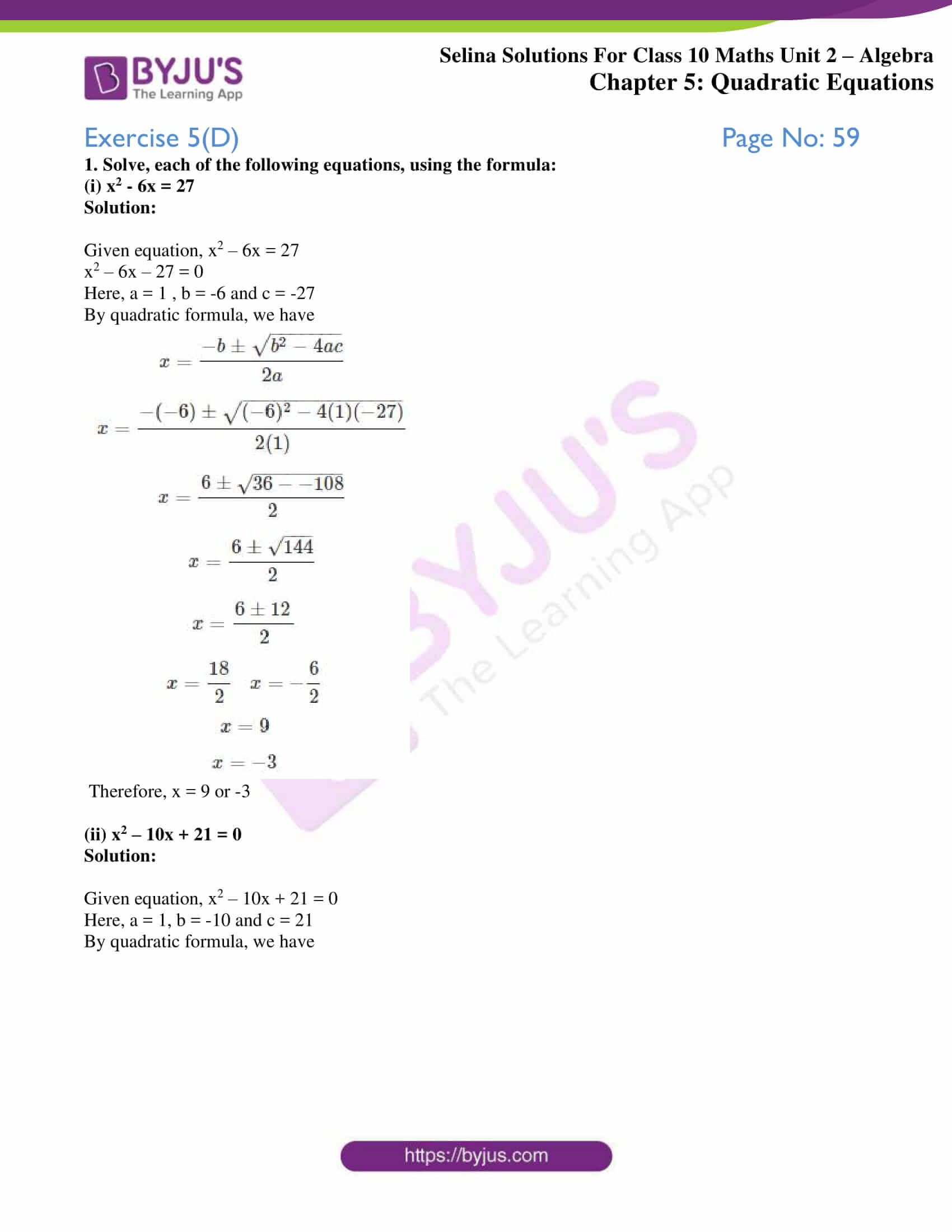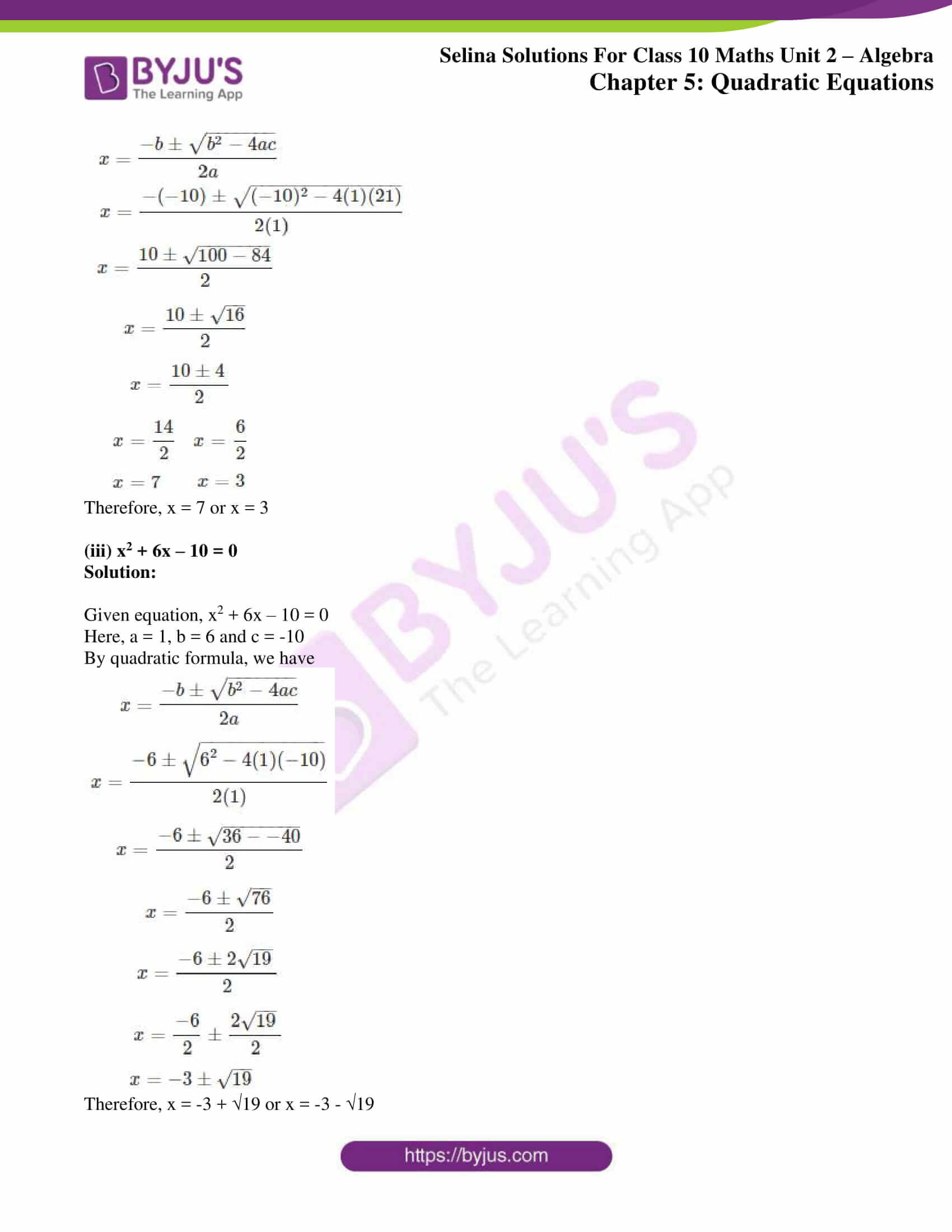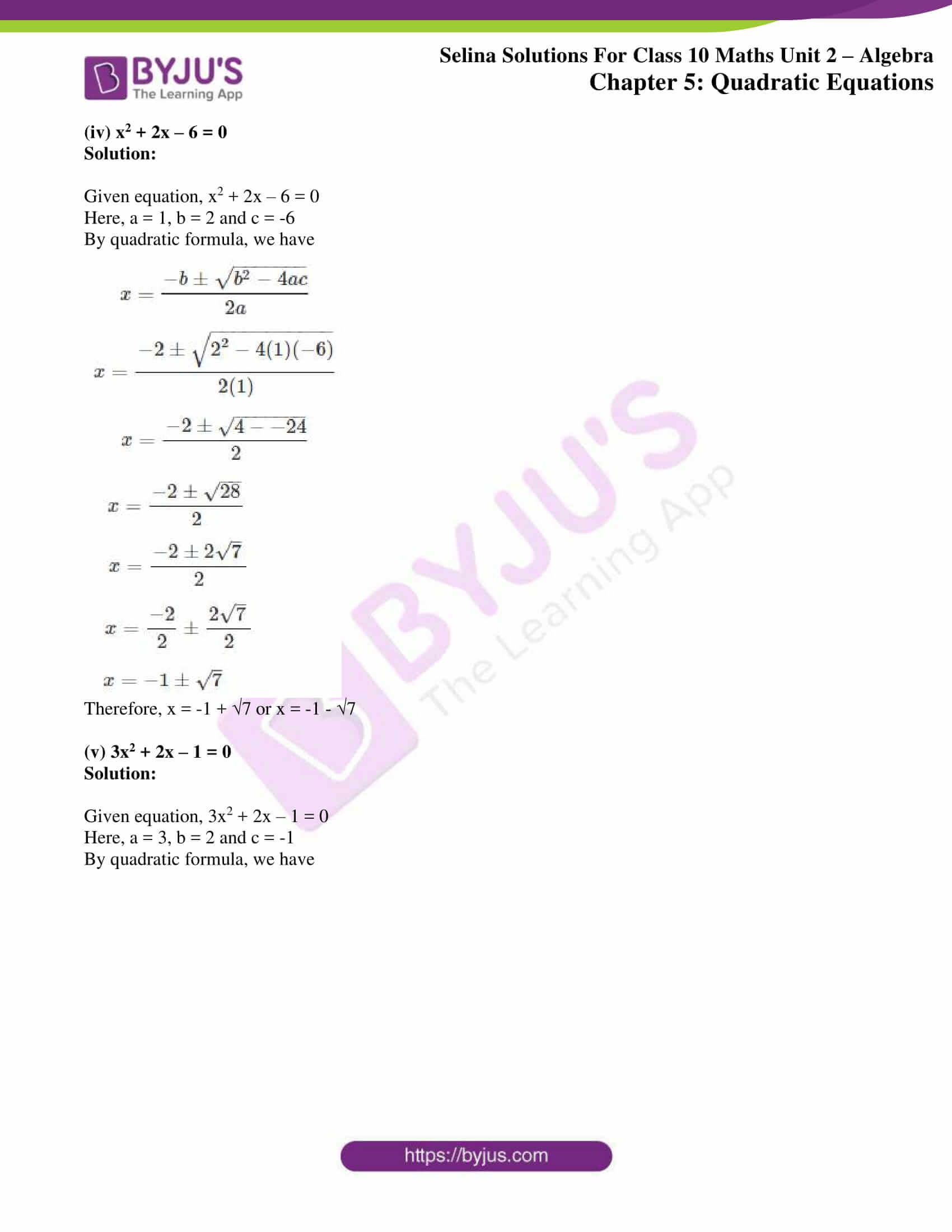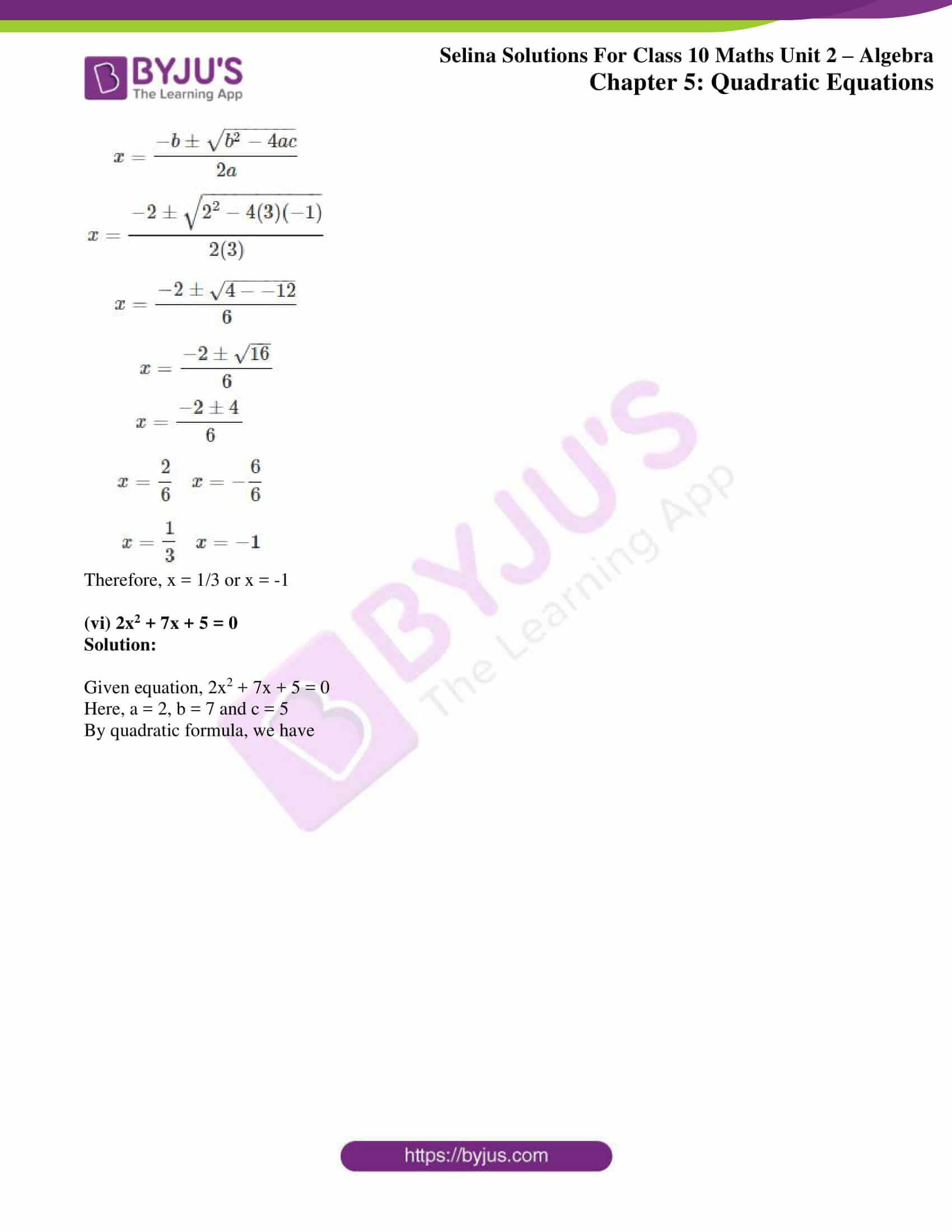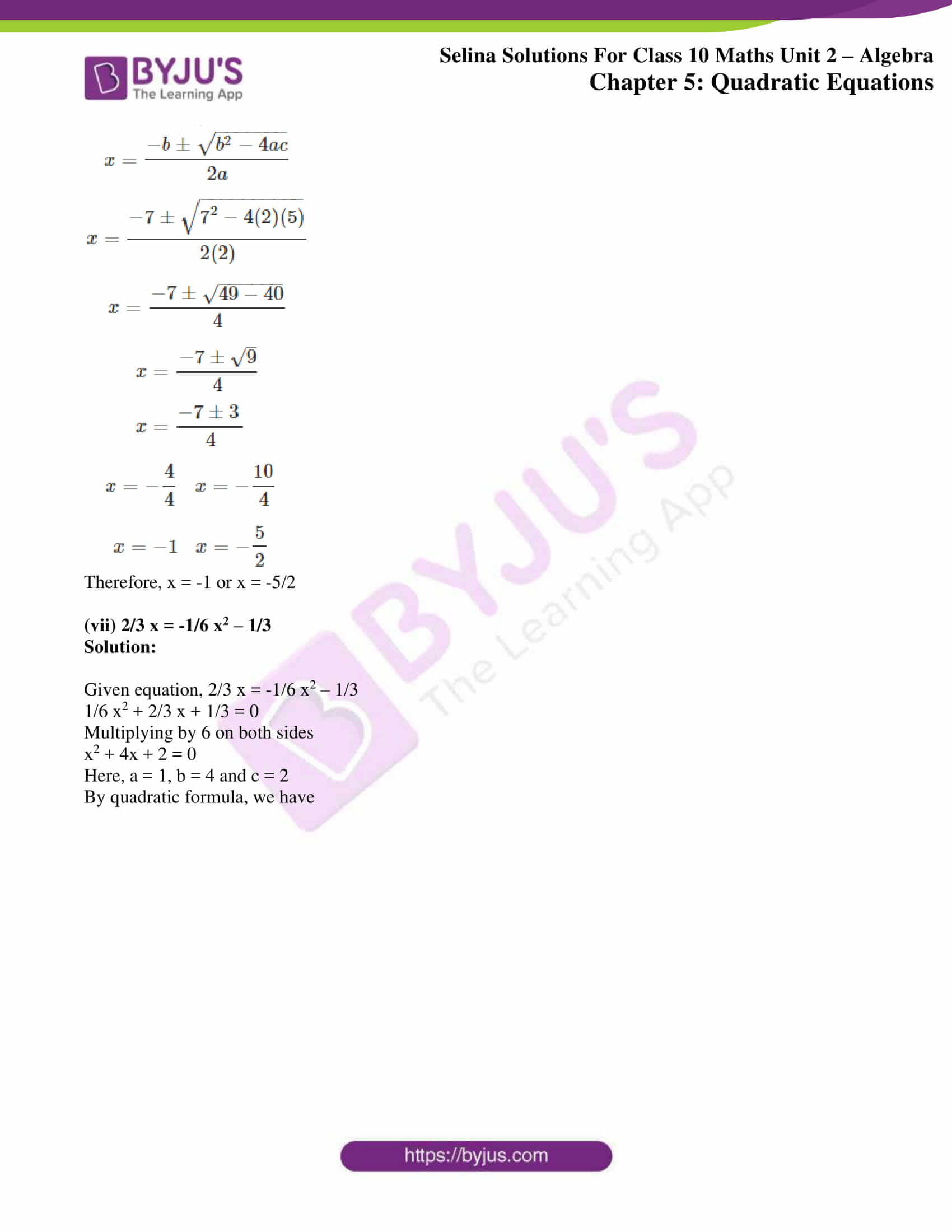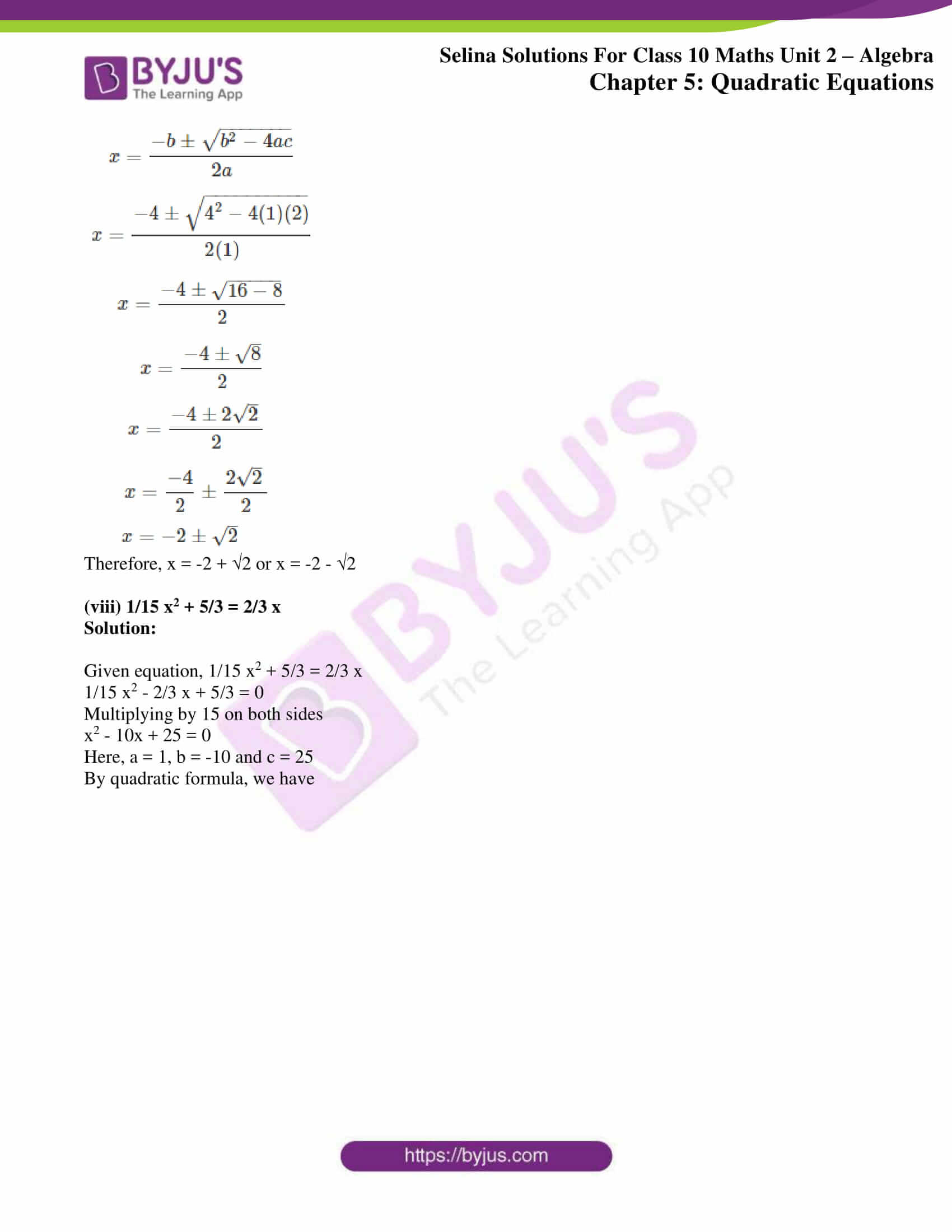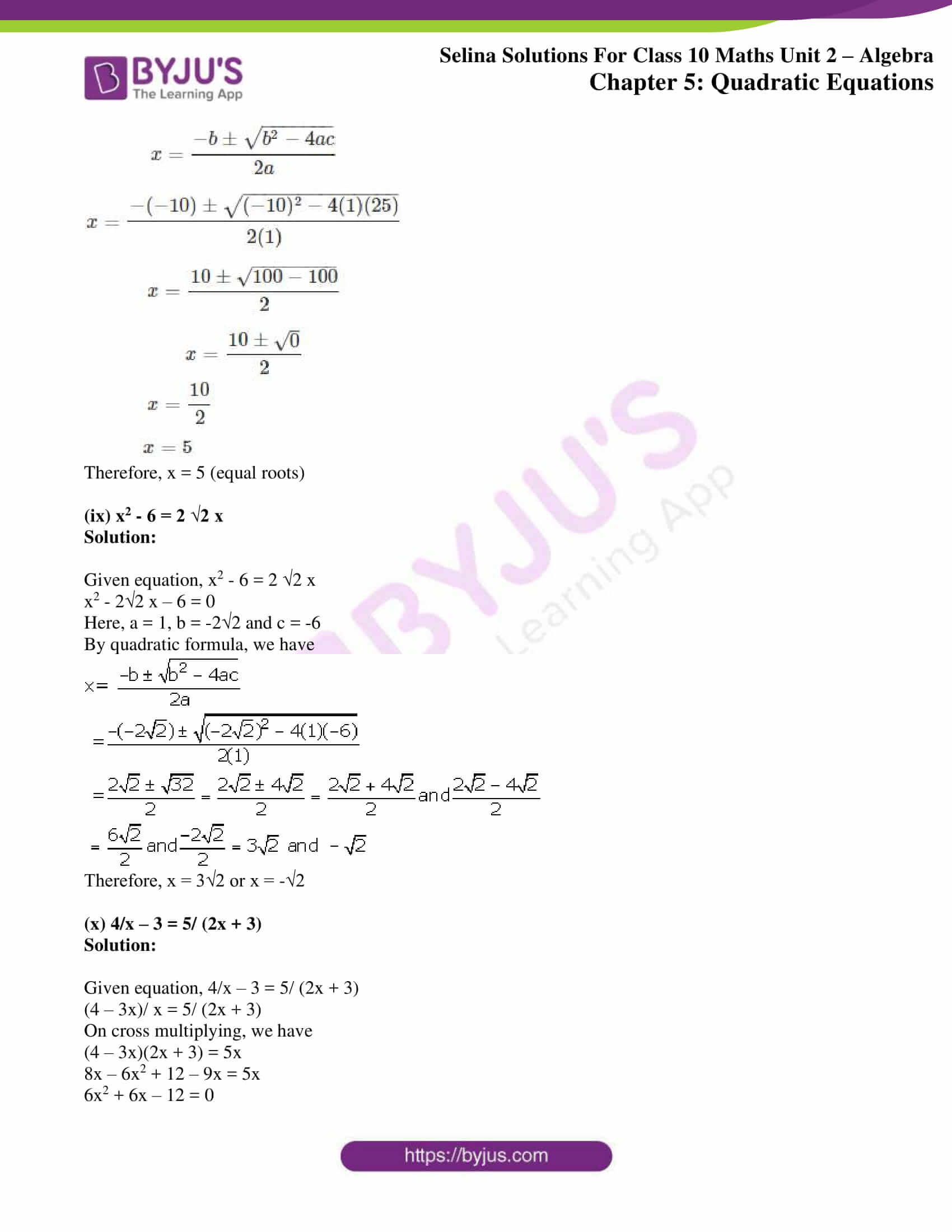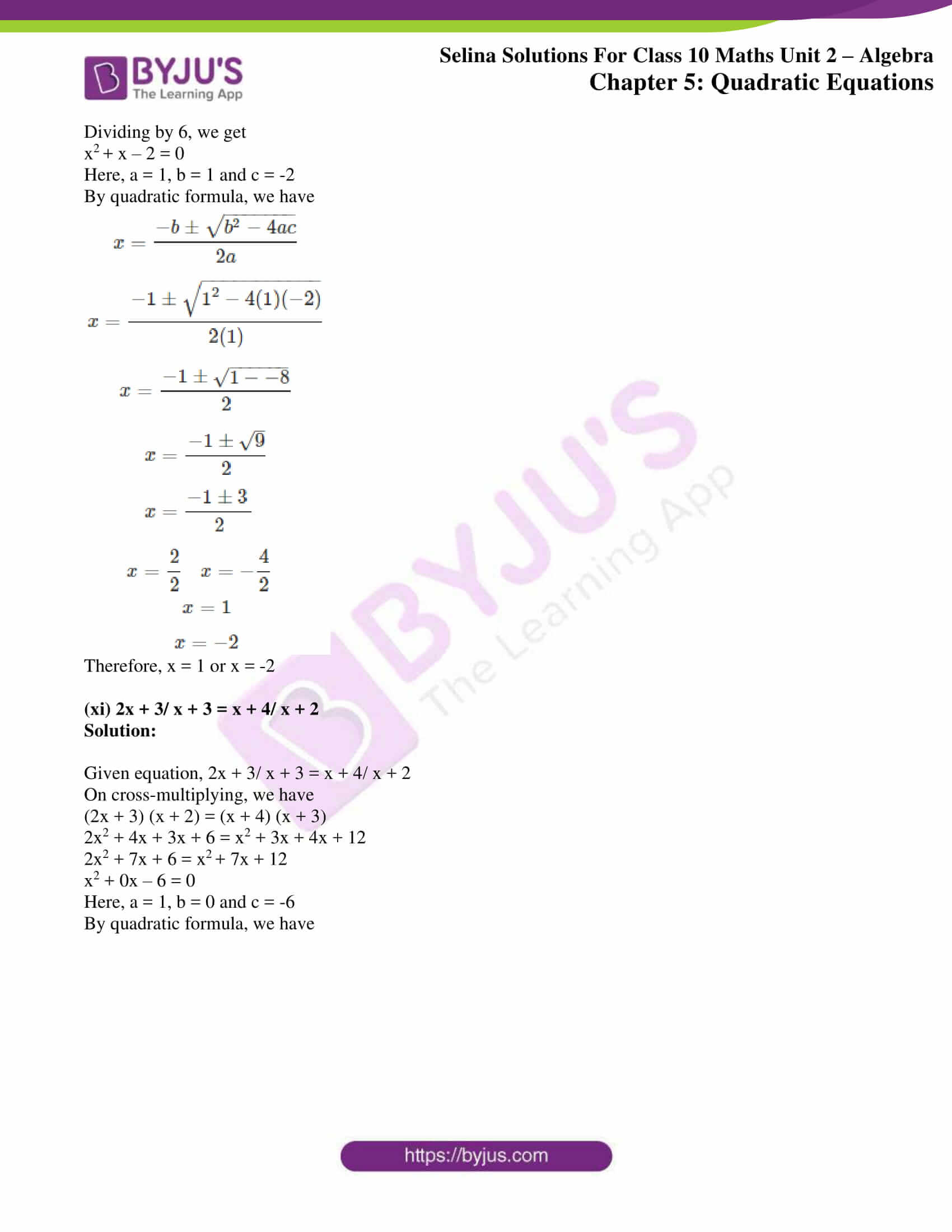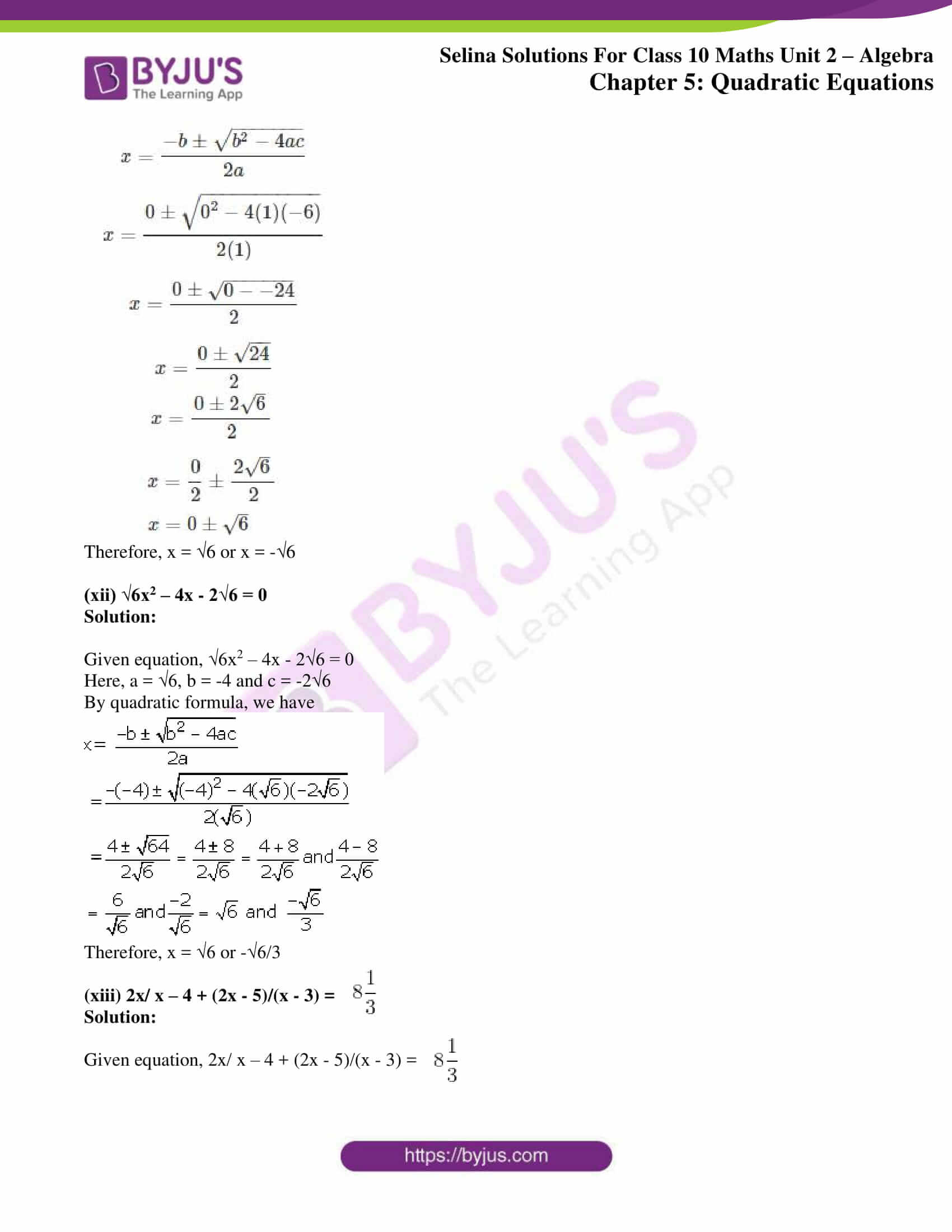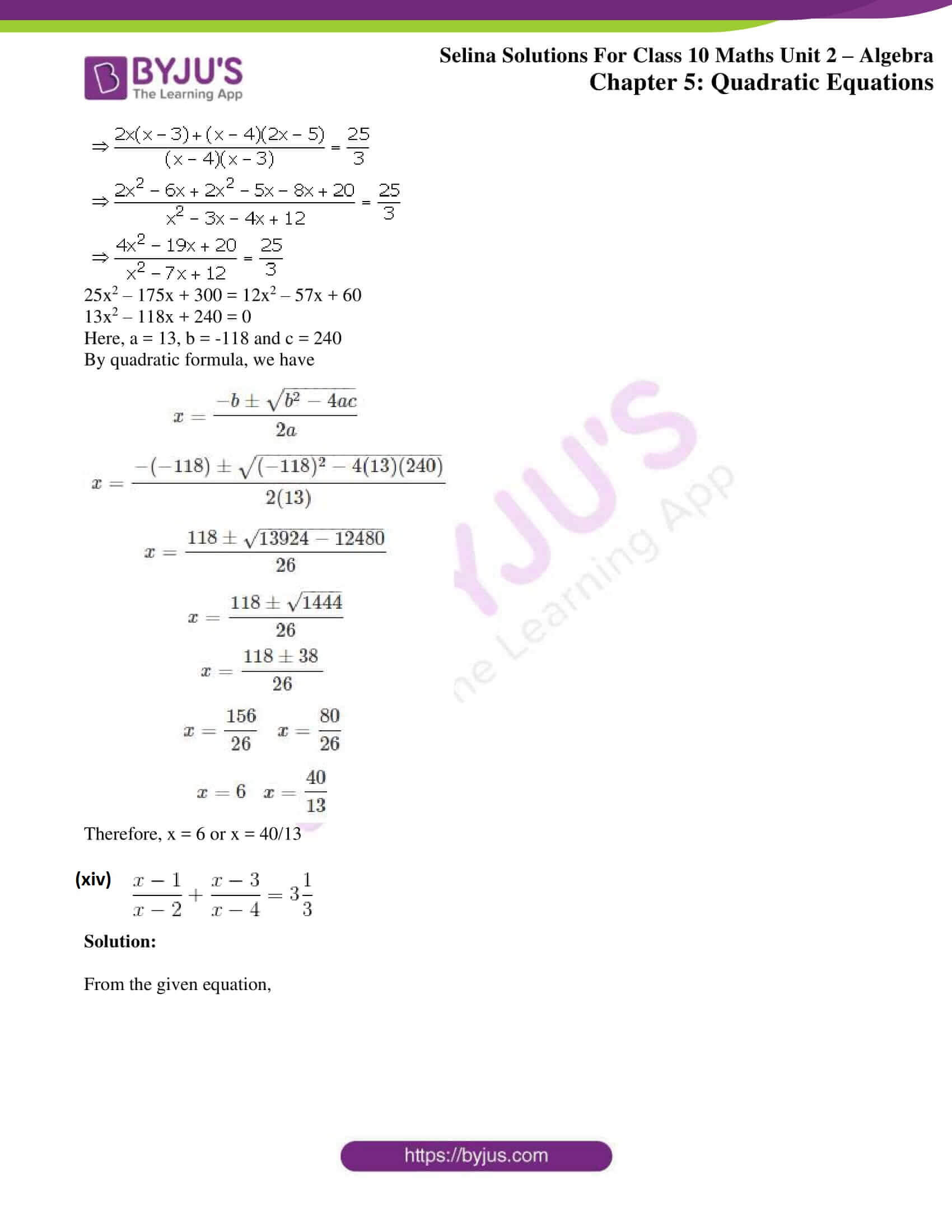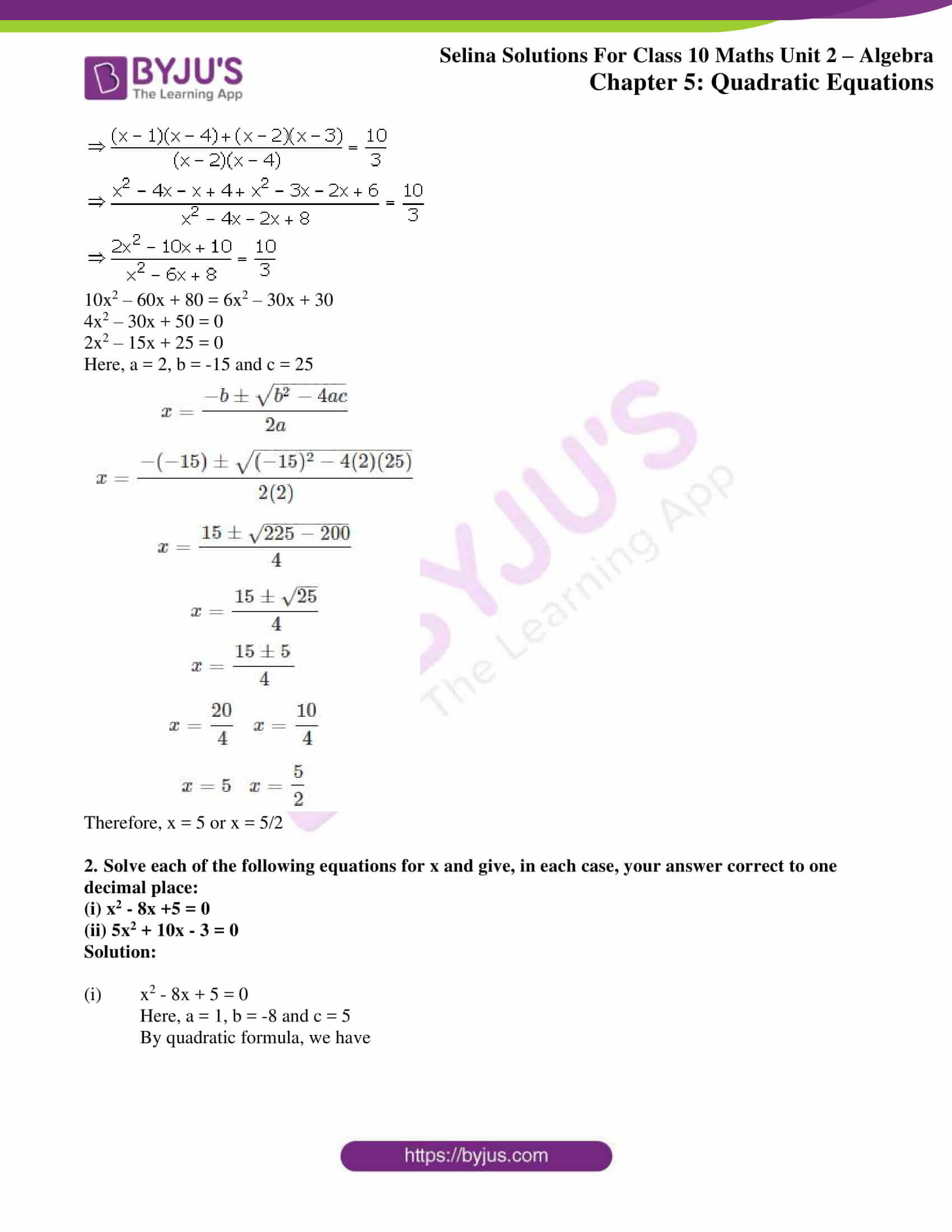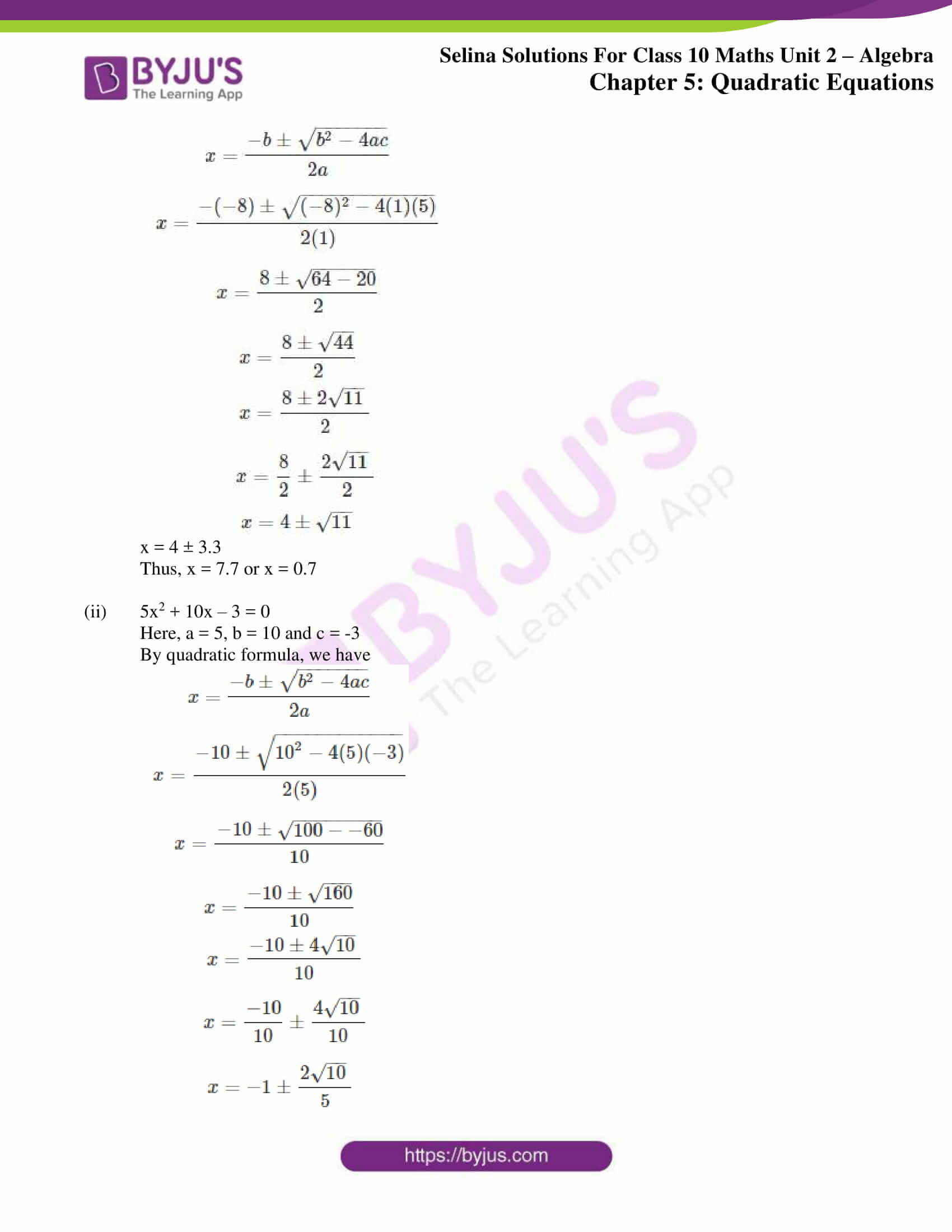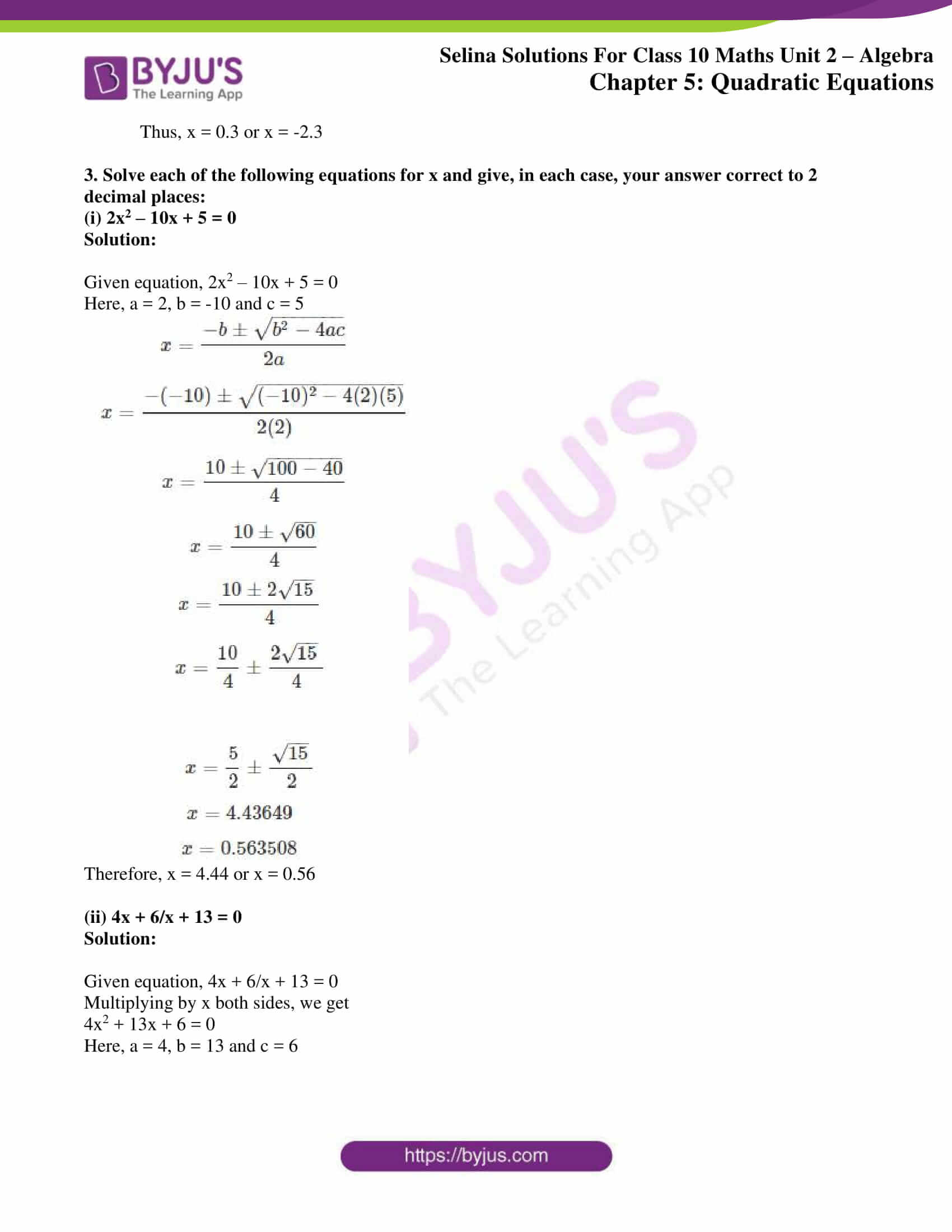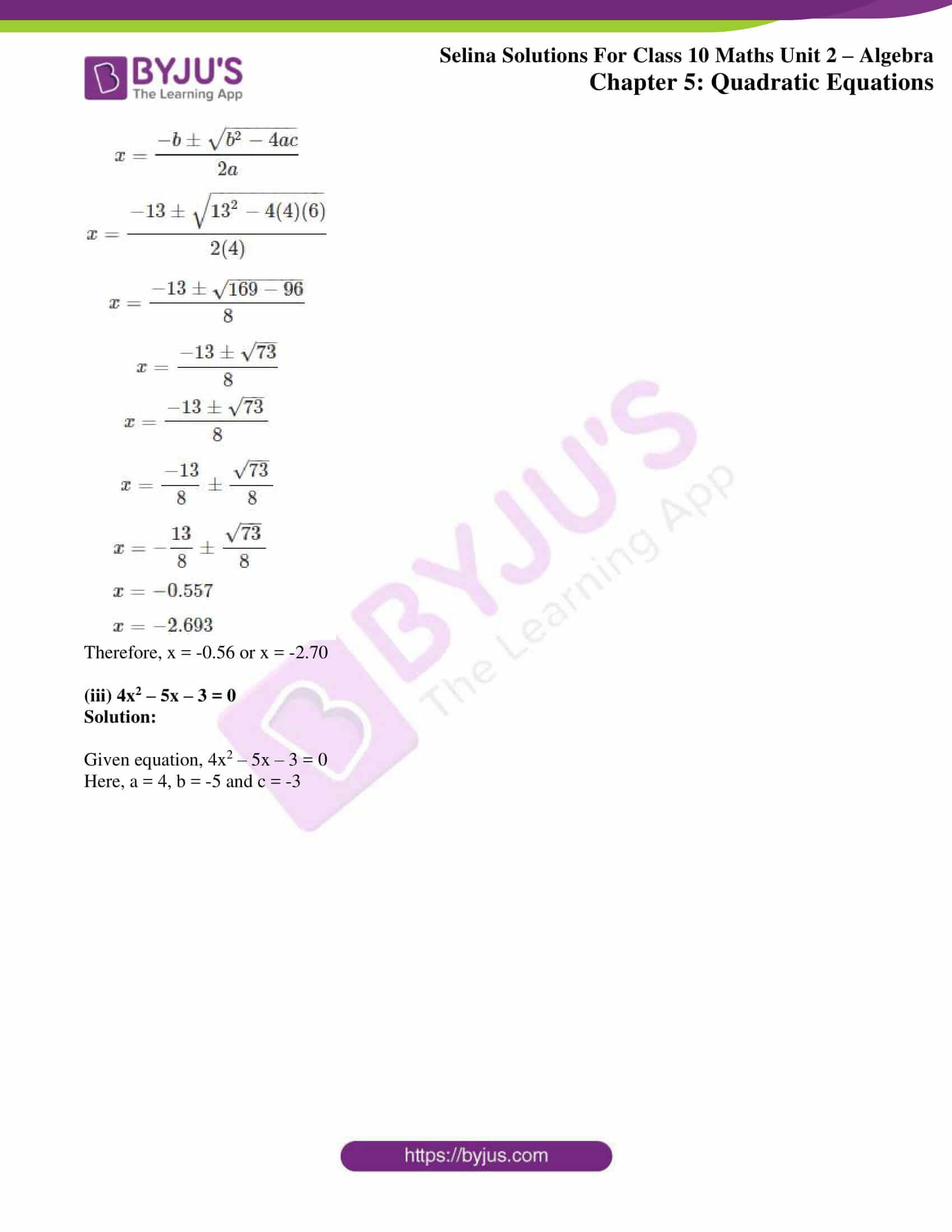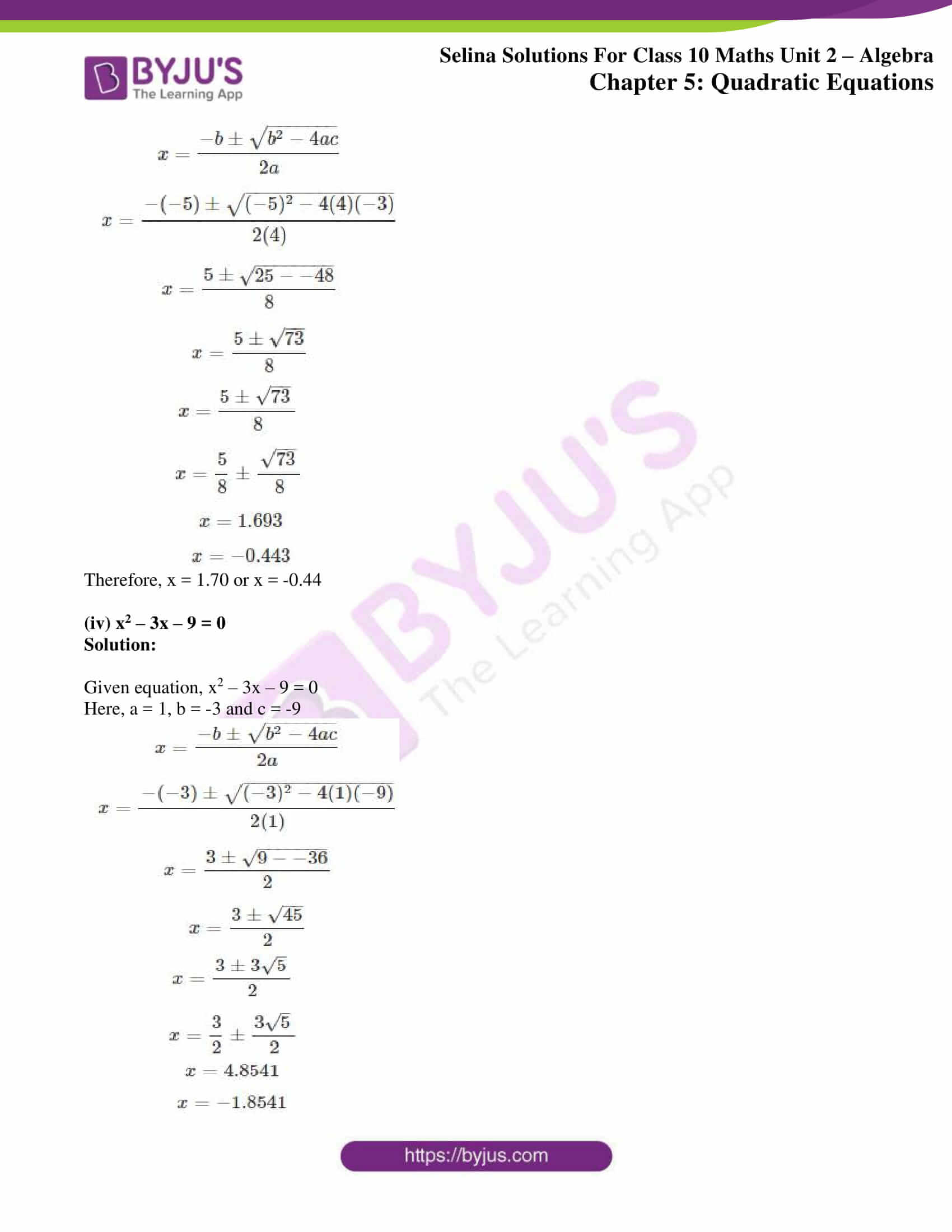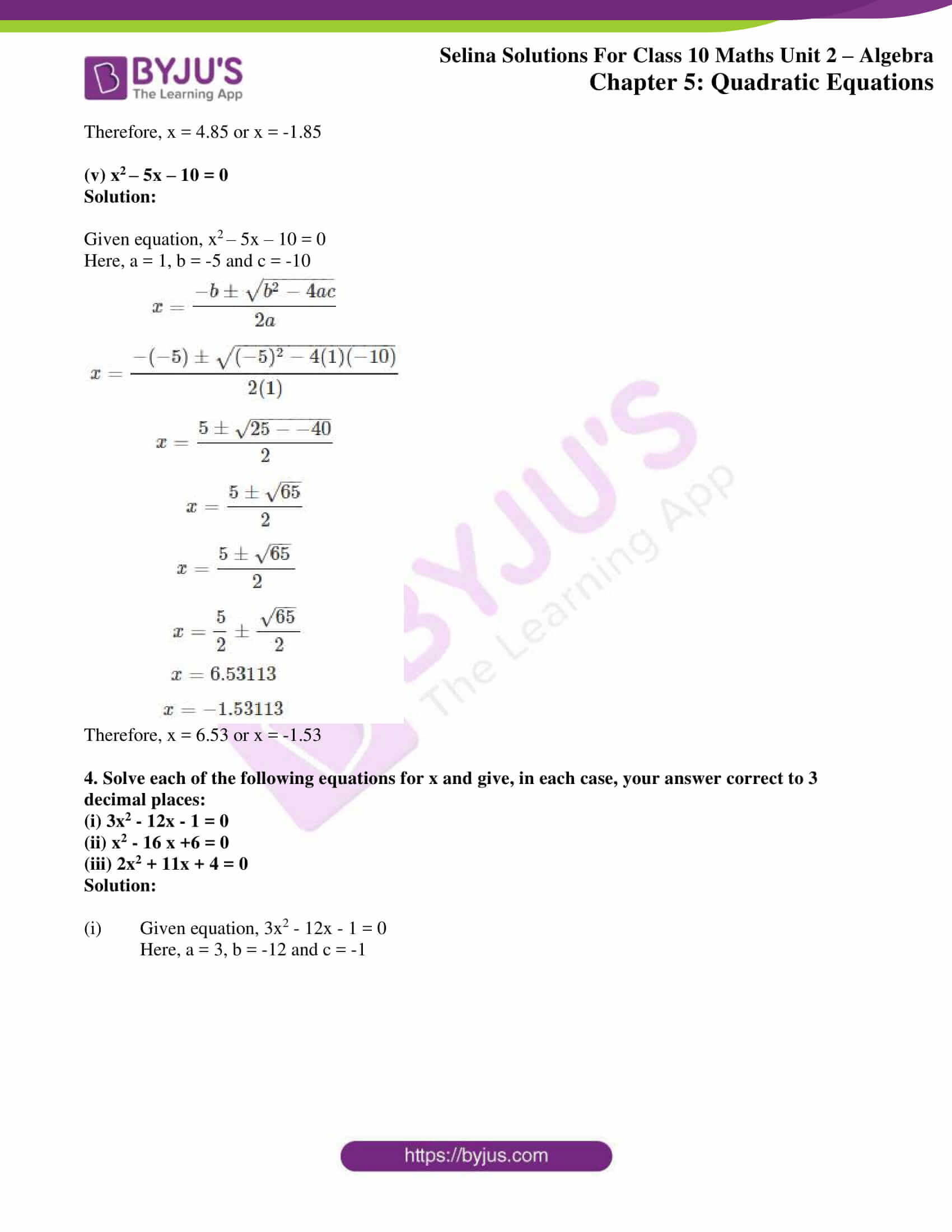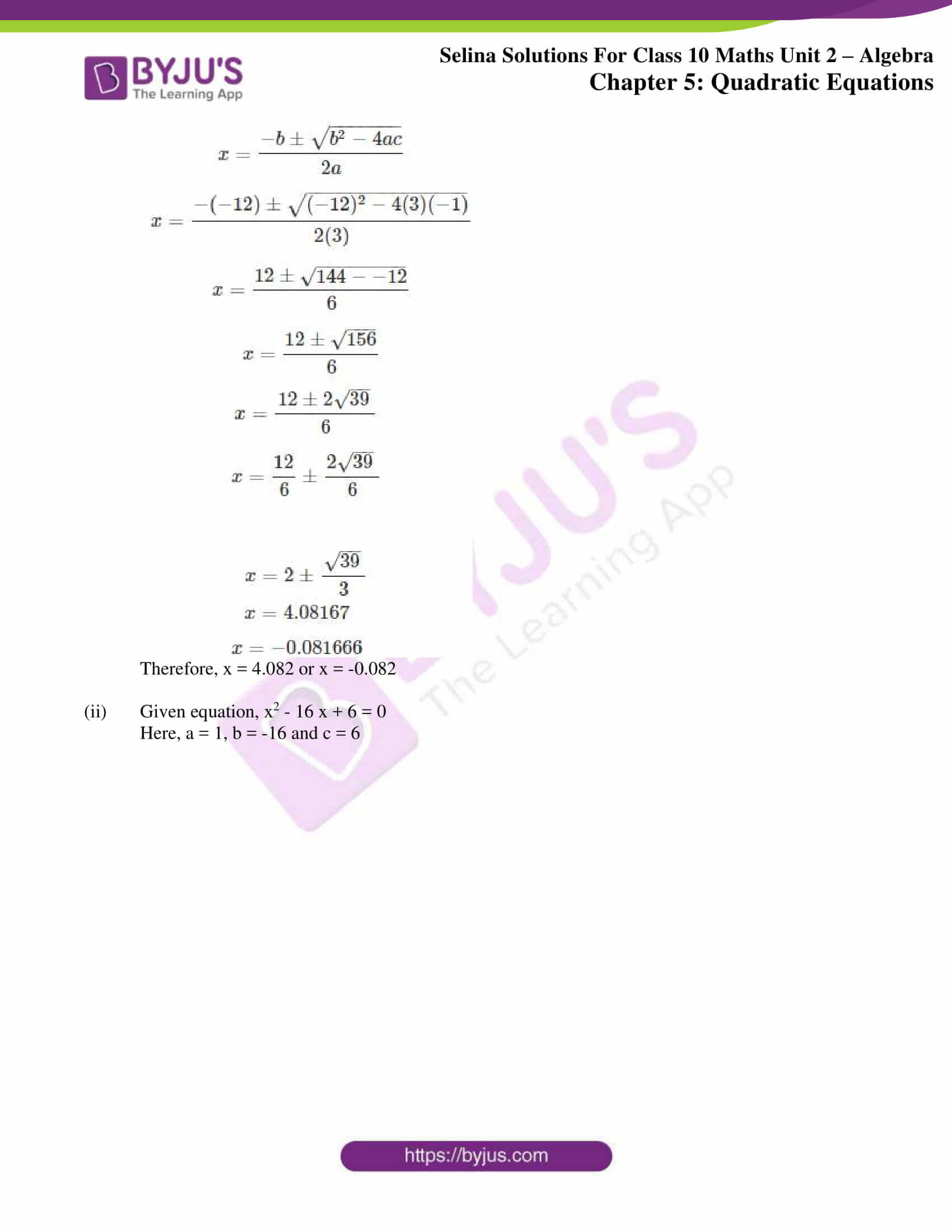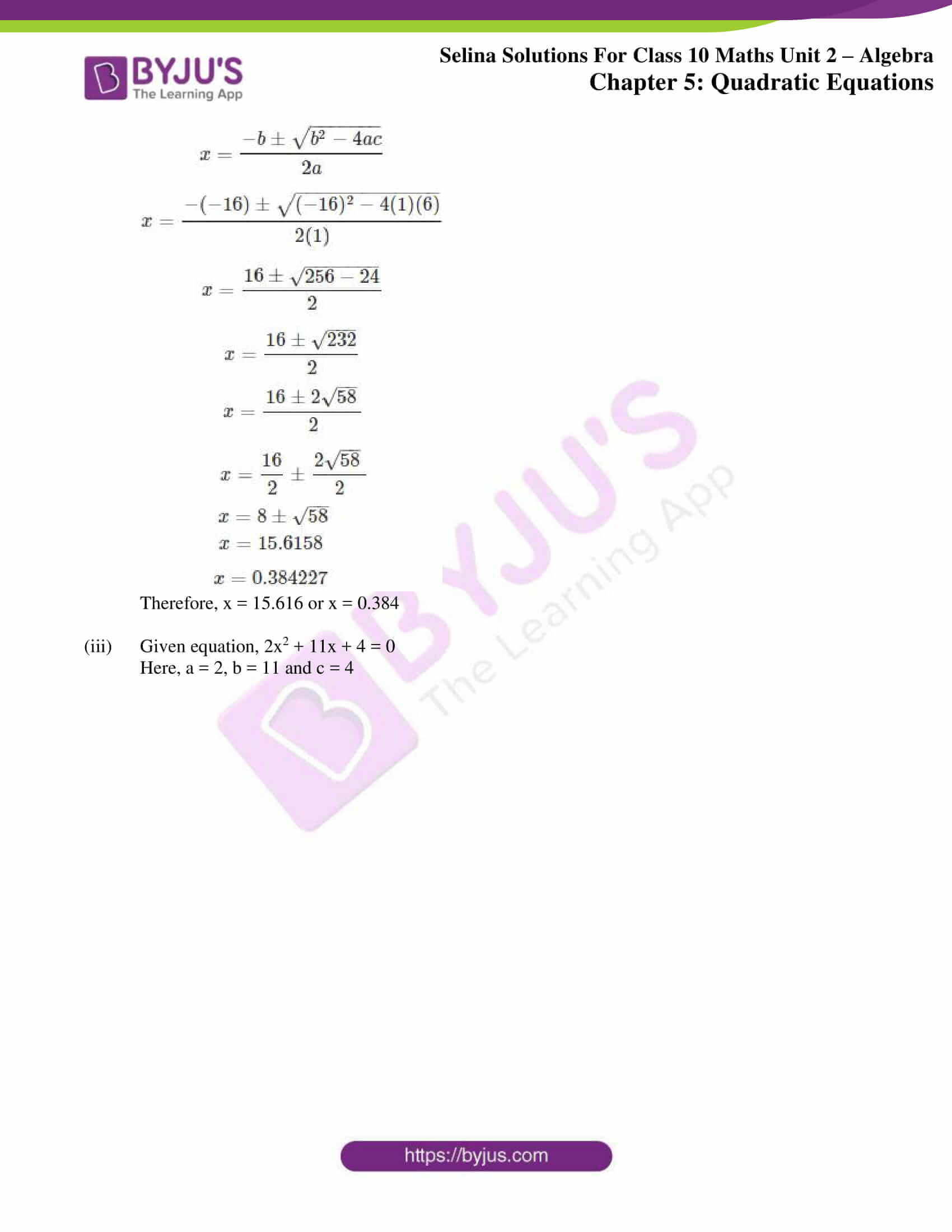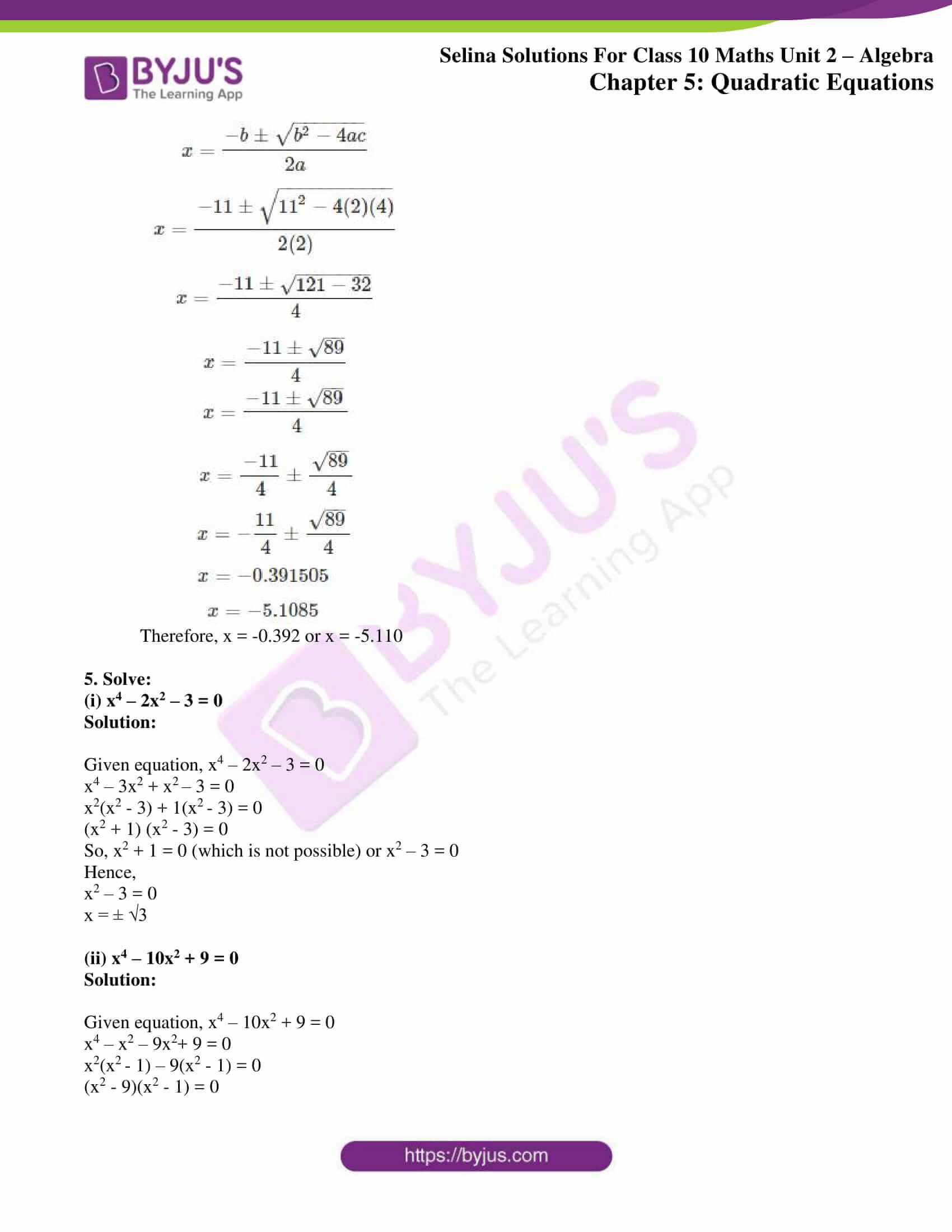### Access other exercises of Selina Solutions Concise Maths Class 10 Chapter 5 Quadratic Equations

Exercise 5(A) Solutions

Exercise 5(B) Solutions

Exercise 5(C) Solutions

Exercise 5(E) Solutions

Exercise 5(F) Solutions

### Access Selina Solutions Concise Maths Class 10 Chapter 5 Quadratic Equations Exercise 5(D)

1. Solve, each of the following equations, using the formula:

(i) x2 – 6x = 27

Solution:

Given equation, x2 – 6x = 27

x2 – 6x – 27 = 0

Here, a = 1 , b = -6 and c = -27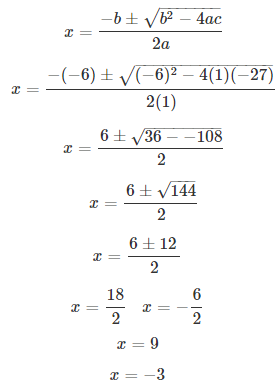Therefore, x = 9 or -3

(ii) x2 – 10x + 21 = 0

Solution:

Given equation, x2 – 10x + 21 = 0

Here, a = 1, b = -10 and c = 21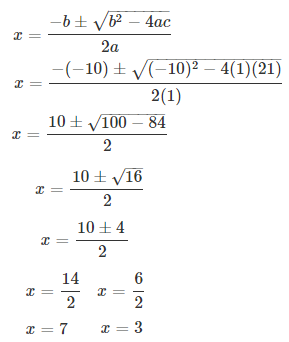Therefore, x = 7 or x = 3

(iii) x2 + 6x – 10 = 0

Solution:

Given equation, x2 + 6x – 10 = 0

Here, a = 1, b = 6 and c = -10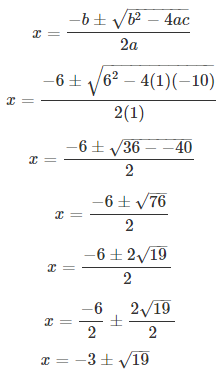Therefore, x = -3 + √19 or x = -3 – √19

(iv) x2 + 2x – 6 = 0

Solution:

Given equation, x2 + 2x – 6 = 0

Here, a = 1, b = 2 and c = -6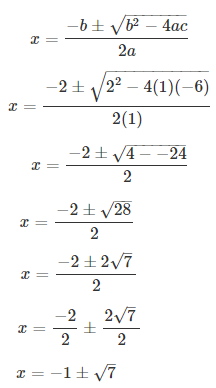Therefore, x = -1 + √7 or x = -1 – √7

(v) 3x2 + 2x – 1 = 0

Solution:

Given equation, 3x2 + 2x – 1 = 0

Here, a = 3, b = 2 and c = -1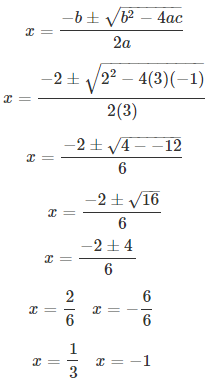Therefore, x = 1/3 or x = -1

(vi) 2x2 + 7x + 5 = 0

Solution:

Given equation, 2x2 + 7x + 5 = 0

Here, a = 2, b = 7 and c = 5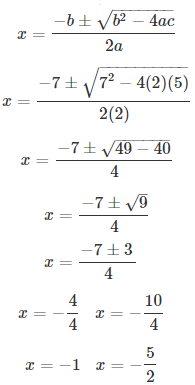Therefore, x = -1 or x = -5/2

(vii) 2/3 x = -1/6 x2 – 1/3

Solution:

Given equation, 2/3 x = -1/6 x2 – 1/3

1/6 x2 + 2/3 x + 1/3 = 0

Multiplying by 6 on both sides

x2 + 4x + 2 = 0

Here, a = 1, b = 4 and c = 2Therefore, x = -2 + √2 or x = -2 – √2

(viii) 1/15 x2 + 5/3 = 2/3 x

Solution:

Given equation, 1/15 x2 + 5/3 = 2/3 x

1/15 x2 – 2/3 x + 5/3 = 0

Multiplying by 15 on both sides

x2 – 10x + 25 = 0

Here, a = 1, b = -10 and c = 25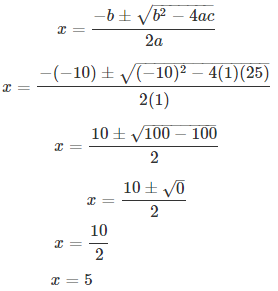Therefore, x = 5 (equal roots)

(ix) x2 – 6 = 2 √2 x

Solution:

Given equation, x2 – 6 = 2 √2 x

x2 – 2√2 x – 6 = 0

Here, a = 1, b = -2√2 and c = -6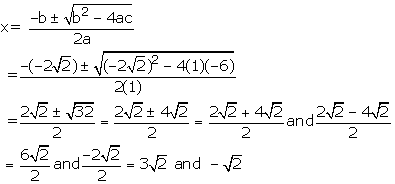Therefore, x = 3√2 or x = -√2

(x) 4/x – 3 = 5/ (2x + 3)

Solution:

Given equation, 4/x – 3 = 5/ (2x + 3)

(4 – 3x)/ x = 5/ (2x + 3)

On cross multiplying, we have

(4 – 3x)(2x + 3) = 5x

8x – 6x2 + 12 – 9x = 5x

6x2 + 6x – 12 = 0

Dividing by 6, we get

x2 + x – 2 = 0

Here, a = 1, b = 1 and c = -2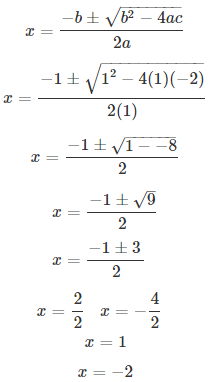Therefore, x = 1 or x = -2

(xi) 2x + 3/ x + 3 = x + 4/ x + 2

Solution:

Given equation, 2x + 3/ x + 3 = x + 4/ x + 2

On cross-multiplying, we have

(2x + 3) (x + 2) = (x + 4) (x + 3)

2x2 + 4x + 3x + 6 = x2 + 3x + 4x + 12

2x2 + 7x + 6 = x2 + 7x + 12

x2 + 0x – 6 = 0

Here, a = 1, b = 0 and c = -6

Therefore, x = √6 or x = -√6

(xii) √6x2 – 4x – 2√6 = 0

Solution:

Given equation, √6x2 – 4x – 2√6 = 0

Here, a = √6, b = -4 and c = -2√6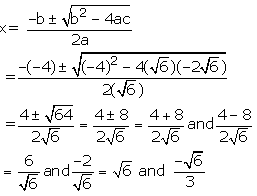Therefore, x = √6 or -√6/3

(xiii) 2x/ x – 4 + (2x – 5)/(x – 3) =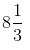Solution:

Given equation, 2x/ x – 4 + (2x – 5)/(x – 3) =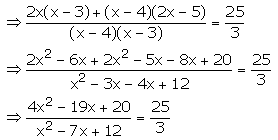25x2 – 175x + 300 = 12x2 – 57x + 60

13x2 – 118x + 240 = 0

Here, a = 13, b = -118 and c = 240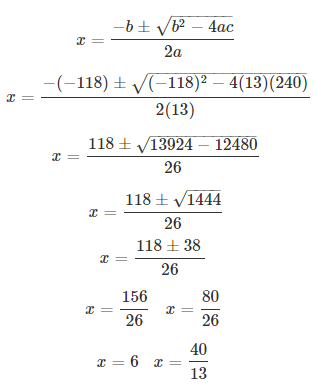Therefore, x = 6 or x = 40/13

(xiv)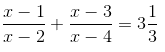Solution:

From the given equation,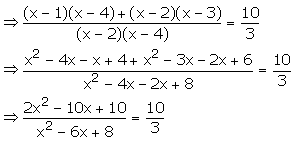10x2 – 60x + 80 = 6x2 – 30x + 30

4x2 – 30x + 50 = 0

2x2 – 15x + 25 = 0

Here, a = 2, b = -15 and c = 25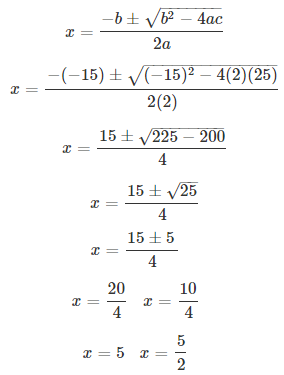Therefore, x = 5 or x = 5/2

2. Solve each of the following equations for x and give, in each case, your answer correct to one decimal place:

(i) x2 – 8x +5 = 0

(ii) 5x2 + 10x – 3 = 0

Solution:

(i) x2 – 8x + 5 = 0

Here, a = 1, b = -8 and c = 5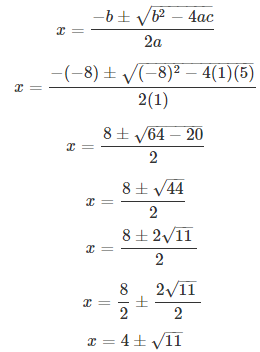x = 4 ± 3.3

Thus, x = 7.7 or x = 0.7

(ii) 5x2 + 10x – 3 = 0

Here, a = 5, b = 10 and c = -3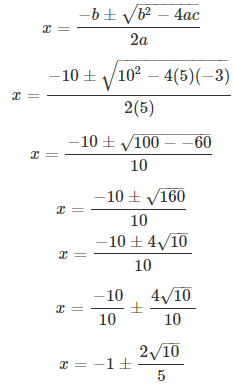Thus, x = 0.3 or x = -2.3

3. Solve each of the following equations for x and give, in each case, your answer correct to 2 decimal places:

(i) 2x2 – 10x + 5 = 0

Solution:

Given equation, 2x2 – 10x + 5 = 0

Here, a = 2, b = -10 and c = 5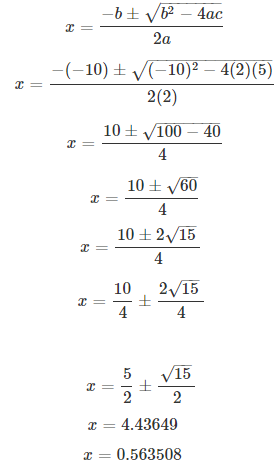Therefore, x = 4.44 or x = 0.56

(ii) 4x + 6/x + 13 = 0

Solution:

Given equation, 4x + 6/x + 13 = 0

Multiplying by x both sides, we get

4x2 + 13x + 6 = 0

Here, a = 4, b = 13 and c = 6Therefore, x = -0.56 or x = -2.70

(iii) 4x2 – 5x – 3 = 0

Solution:

Given equation, 4x2 – 5x – 3 = 0

Here, a = 4, b = -5 and c = -3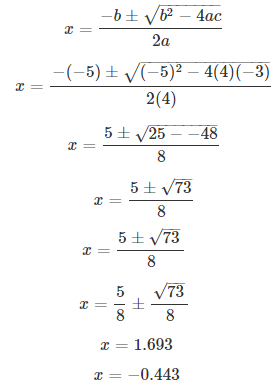Therefore, x = 1.70 or x = -0.44

(iv) x2 – 3x – 9 = 0

Solution:

Given equation, x2 – 3x – 9 = 0

Here, a = 1, b = -3 and c = -9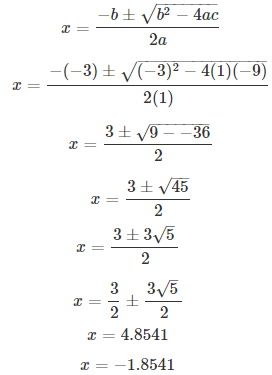Therefore, x = 4.85 or x = -1.85

(v) x2 – 5x – 10 = 0

Solution:

Given equation, x2 – 5x – 10 = 0

Here, a = 1, b = -5 and c = -10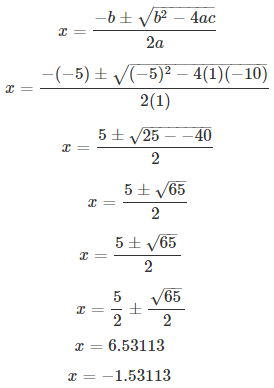Therefore, x = 6.53 or x = -1.53

4. Solve each of the following equations for x and give, in each case, your answer correct to 3 decimal places:

(i) 3x2 – 12x – 1 = 0

(ii) x2 – 16 x +6 = 0

(iii) 2x2 + 11x + 4 = 0

Solution:

(i) Given equation, 3x2 – 12x – 1 = 0

Here, a = 3, b = -12 and c = -1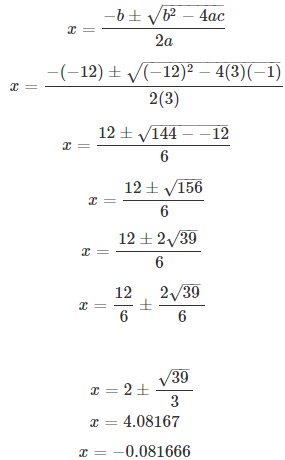Therefore, x = 4.082 or x = -0.082

(ii) Given equation, x2 – 16 x + 6 = 0

Here, a = 1, b = -16 and c = 6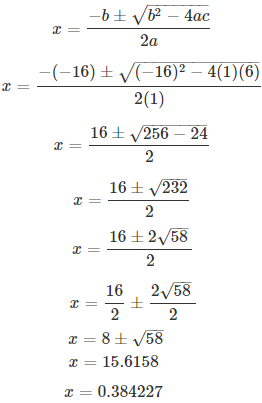Therefore, x = 15.616 or x = 0.384

(iii) Given equation, 2x2 + 11x + 4 = 0

Here, a = 2, b = 11 and c = 4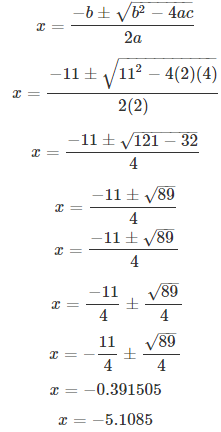Therefore, x = -0.392 or x = -5.110

5. Solve:

(i) x4 – 2x2 – 3 = 0

Solution:

Given equation, x4 – 2x2 – 3 = 0

x4 – 3x2 + x2 – 3 = 0

x2(x2 – 3) + 1(x2 – 3) = 0

(x2 + 1) (x2 – 3) = 0

So, x2 + 1 = 0 (which is not possible) or x2 – 3 = 0

Hence,

x2 – 3 = 0

x = ± √3

(ii) x4 – 10x2 + 9 = 0

Solution:

Given equation, x4 – 10x2 + 9 = 0

x4 – x2 – 9x2+ 9 = 0

x2(x2 – 1) – 9(x2 – 1) = 0

(x2 – 9)(x2 – 1) = 0

So, we have

x2 – 9 = 0 or x2 – 1 = 0

Hence,

x = ± 3 or x = ± 1

#### 1 Comment

1. Harshi

Hiii nice …thnx a lot for it